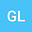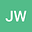The Probability of Events for Parabolic Equations
••• Guangying Lv,
• Jinlong Wei
Guangying Lv
Henan University
Author ProfileJinlong Wei
Zhongnan University of Economics and Law
Author Profile## Abstract

In this short paper, we focus on the blowup phenomenon of stochastic parabolic equations. We first discuss the probability of the event that the solutions keep positive. Then, the blowup phenomenon in the whole space is considered. The probability of the event that the solutions blow up in finite time is given. Lastly, we obtain the probability of the event that blowup time of stochastic parabolic equations larger than or less than the deterministic case.